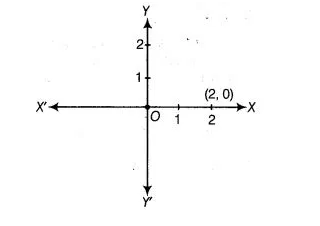# Write whether the following statements are true or false?`
Question:

Write whether the following statements are true or false? Justify your answer.

(i) Point (3, 0) lies in the first quadrant.

(ii) Points (1 -1) and (-1, 1) lie in the same quadrant.

(iii) The coordinates of a point whose ordinate is – ½ and abscissa is 1 are (-½ ,1).

(iv) A point lies on Y-axis at a distance of 2 units from the X-axis. Its coordinates are (2, 0).

(v) (-1,7) is a point in the second quadrant.

Solution:

(i) False, since the ordinate of the point (3, 0) is zero. So, the point lies on X-axis.

(ii) False, because in point (1, -1) x-coordinate is positive and y-coordinate is negative, so it lies in IV quadrant and in (-1,1), x-coordinate is

negative and y-coordinate is positive, so it lies in II quadrant.

(iii) False, because stating the coordinates of a point abscissa comes first and then ordinate (1,-½).

(iv) False, because point (2, 0) lies on X-axis whose distance from Y-axis is 2 units.(v) True, because in a point (-1, 7) abscissa is negative and ordinate is positive.